Click to Chat

1800-1023-196

+91-120-4616500

CART 0

• 0

MY CART (5)

Use Coupon: CART20 and get 20% off on all online Study Material

ITEM
DETAILS
MRP
DISCOUNT
FINAL PRICE
Total Price: Rs.

There are no items in this cart.
Continue Shopping```Determinants- Definitions & Properties

Definitions of Determinantes & Properties

Consider the equations a1x + b1y = 0, a2x + b2y = 0. These give -a1/b1 = y/x = - a2/b2 => a1/b1 = a2/b2

=> a1b2 - a2b1 = 0.

We express this eliminant as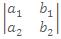= 0.

The expressionis called a determinant of order two, and equals a1b2-a2b1.

A determinant of order three consisting of 3 rows and 3 columns is written asand is equal to a1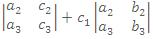= a1(b2c3-c2b3)-b1(a2c3-c

2a3)+c1(a2b3-b2a3).

The numbers ai, bi, ci (i = 1 2, 3,) are called the elements of the determinant.

The determinant, obtained by deleting the ith row and the jth column is called the minor of the element at ith row and jth column. The cofactor of this element is (-1)i+j (minor). Note that: Δ ==a1A1 + b1B1 + c1C1

where A1, B1 and C1 are the cofactors of a1, b1 and c1 respectively.

We can expand the determinant through any row or column. It means we can write Δ = a2A2 + b2B2 + c2C2 or Δ = a1A1 + a2A2 + a3A3 etc.

Also a1A2 + b1B2 + c1C2 = 0

=> aiAj + biBj + ciCj       = Δ   if      i = j,

= 0   if      i ≠ j.

These results are true for determinants of any order.

​Properties of Determinants

(i) If rows be changed into columns and columns into the rows, then the values of the determinant remains unaltered.

(ii) If any two row (or columns) of a determinant are interchanged, the resulting determinant is the negative of the original determinant.

(iii) If two rows (or two columns) in a determinant have corresponding elements that are equal, the value of determinant is equal to zero.

(iv) If each element in a row (or column) of a determinant is written as the sum of two or more terms then the determinant can be written as the sum of two or more determinants.

Illustration:

Without expanding, show thatSolution:

L.H.S. =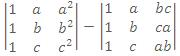On the second determinant operate aR1, bR2, cR3 and then take abc common out of C3

=> L.H.S. =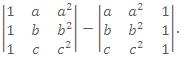.

Interchange C1 and C3 and then C2 and C3

So that L.H.S. =.

(vi) If to each element of a line (row or column) of a determinant be added the equimultiples of the corresponding elements of one or more parallel lines, the determinant remains unaltered

i.e.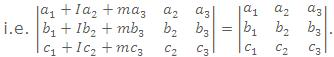.

Illustration:

Evaluate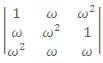where ω is cube root of unit.

Solution:

Applying C1 -> C1 + C2 + C3 we get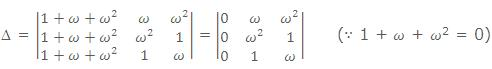= 0.

(vii) If each element in any row (or any column) of determinant is zero, then the value of determinant is equal to zero.

(viii) If a determinant D vanishes for x = a, then (x - a) is a factor of D, In other words, if two rows (or two columns) become identical for x = a, then (x-a) is a factor of D.

In general, if r rows (or r columns) become identical when a is substituted for x, then (x-)r-1 is a factor of D.
IIT JEE study material is available online free of cost at askIITians.com. Study Set Theory, Functions and a number of topics of Algebra at askIITians website and be a winner. We offer numerous live online courses for IIT JEE preparation - you do not need to travel anywhere any longer - just sit at your home and study for IIT JEE live online with askIITians.com

To read more, Buy study materials of Matrices and Determinants comprising study notes, revision notes, video lectures, previous year solved questions etc. Also browse for more study materials on Mathematics here.
```### Course Features

• 731 Video Lectures
• Revision Notes
• Previous Year Papers
• Mind Map
• Study Planner
• NCERT Solutions
• Discussion Forum
• Test paper with Video Solution